Worksheets and No Prep Teaching Resources
Math Worksheets

# Ordering Numbers Worksheets

Order is a basic element of mathematics, so ordering numbers is an essential skill. These comprehensive worksheets take students through the basics of ordering whole numbers to 999 in creative ways. Then students can master the more challenging concepts of ordering fractions and decimals with unique approaches that will make these ideas stick in young mathematical brains!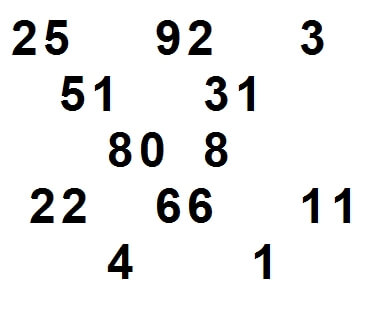Order Numbers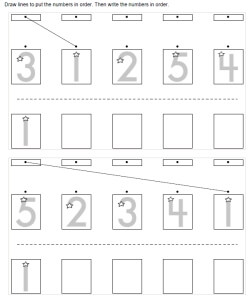Ordering five numbers (numbers 1 to 5) Ordering five numbers (numbers can be from 1 to 9)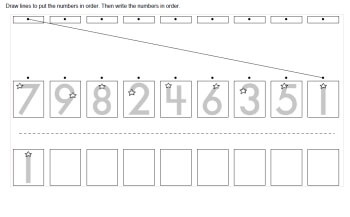Ordering nine numbers (numbers 1 to 9)

Ordering Numbers Math Center - Whole Numbers

Ordering Numbers Math Center - Fractions

Ordering Numbers Math Center - Negative Numbers

Ordering Numbers Math Center - Decimals

Ordering Whole Numbers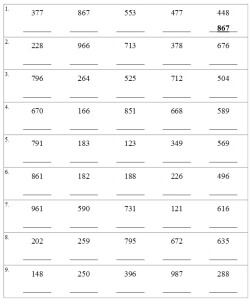Ordering numbers from 0 to 20 Ordering numbers from 0 to 50 Ordering numbers from 0 to 100 Ordering numbers from 100 to 999

Ordering Fractions

Ordering Numbers
Numbers: 0-10
Fill in the missing numbers (Just before, after, and between)
Greater than, less than, and equal to (circle the words)
Circle the larger number
Circle the smaller number
Order 3 numbers (from smallest to largest)
Order 3 numbers (from largest to smallest)
Order 4 numbers
Ordering Mixed Review

Numbers: 11-20
Fill in the missing numbers (Just before, after, and between)
Greater than, less than, and equal to (circle the words)
Circle the larger number
Circle the smaller number
Order 3 numbers (from smallest to largest)
Order 3 numbers (from largest to smallest)
Order 4 numbers
Ordering Mixed Review

Numbers: 0 to 20
Fill in the missing numbers (Just before, after, and between)
Greater than, less than, and equal to (circle the words)
Circle the larger number
Circle the smaller number
Order 3 numbers (from smallest to largest)
Order 3 numbers (from largest to smallest)
Order 4 numbers
Ordering Mixed Review

Numbers: 0 to 50
Fill in the missing numbers (Just before, after, and between)
Greater than, less than, and equal to (circle the words)
Circle the larger number
Circle the smaller number
Order 3 numbers (from smallest to largest)
Order 3 numbers (from largest to smallest)
Order 4 numbers
Ordering Mixed Review

Numbers: 0 to 99
Fill in the missing numbers (Just before, after, and between)
Greater than, less than, and equal to (circle the words)
Circle the larger number
Circle the smaller number
Order 3 numbers (from smallest to largest)
Order 3 numbers (from largest to smallest)
Order 4 numbers
Ordering Mixed Review

Comparing Numbers

Number Lines

Ordering Decimals
Tenths and hundredths: Order decimals
Order decimals (from 6 to 8 numbers each)
Compare decimals and fractions
Order decimals and fractions
Order decimals and fractions (from 7 to 11 numbers each)
Compare decimals and fractions (with numbers greater than 1)
Order decimals and fractions (with numbers greater than 1)
Tenths and Hundredths Mixed Review
Ordering decimals
Compare decimals (including fractions)
Ordering decimals (including fractions)

Ordering Repeating Decimals
Order 3 numbers
Order 7 numbers
Order 7 numbers (includes negatives)

Measurement
Ordering Metric units

Ordering and Counting
 Ordering Animals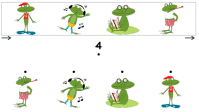Ordering Animals

 Ordering Shapes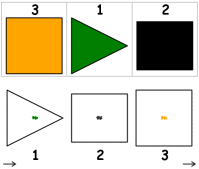Ordering Shapes

Greater and Less Than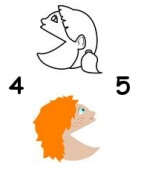Greater and Less Than

Have a suggestion or would like to leave feedback?